# Vitali set

The term Vitali set describes any set obtained by a particular mathematical construction. The construction uses the axiom of choice and its result given by an existence theorem is not uniquely determined. Vitali sets have many important applications in mathematics, most notable being a proof of existence of Lebesgue non-measurable sets in measure theory. The name was given after the Italian mathematician Giuseppe Vitali.

##  Formal construction

We begin by defining the following relation on the real line. Two real numbers x and y are said to be equivalent if and only if the difference x-y is rational. In symbols,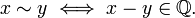$x \sim y \iff x-y \in \mathbb{Q}.$

It is easy to verify that it is in fact an equivalence relation. Thus, it yields a partition of the set of reals into its equivalence classes. By the axiom of choice we can select a representative of each single class. The Vitali set V is defined to be the set of all selected representatives.

Since for any real x the elements of the set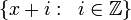$\{ x+i:\;\;i\in \mathbb{Z} \}$ are in the same equivalence class, me may (and do) additionally require that$V\subset [0,1]$ (indeed, x+i belong to [0,1] for some i).

##  Application to measure theory

A Vitali set can not be included in the family of measurable sets for any locally finite translation invariant measure (except for the zero measure). In particular it is not Lebesgue measurable.

More precisely, suppose that a measure μ defined over a σ-algebra Σ of subsets of the real line satisfies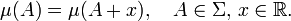$\mu(A) = \mu(A+x),\quad A\in\Sigma,\, x\in \mathbb{R}.$

In particular, this presumes that any translation of any measurable set is measurable. We also assume that$\mu(\mathbb{R}) \ne 0$ and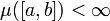$\mu([a,b]) < \infty$ for some (or, equivalently, all) a < b. We will show that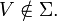$V\notin \Sigma.$

Observe that for any rational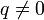$q\not=0$ the sets V+q and V are disjoint. This is because if there is any$x\in (V+q)\cap V$ then at the same time$x-q\in V$ and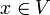$x\in V$. In other words V contains two distinct representatives of the same equivalence class, which contradicts the definition of V.

Let q1,q2,... be an enumeration of the rationals from [-1,1] and define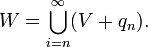$W=\bigcup_{i=n}^\infty (V+q_n).$

Then, clearly$[0,1]\subset W\subset [-1,2].$

Indeed, the second inclusion follows directly from the fact that$V\subset[0,1]$ and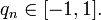$q_n\in[-1,1].$ For the first one, observe that for any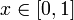$x\in [0,1]$, the class of equivalence of x has its unique representative y in V. Therefore x-y is rational and, by the fact that$V\subset[0,1]$, we have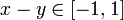$x-y\in [-1,1]$. In other words, xy = qn for some n and so$x\in V+q_n\subset W$.

Now suppose that the set V is measurable (and so are V + qn). Recall that by definition any measure is supposed to be countably additive. It follows that$\mu(W) = \mu (\bigcup_{n=1}^\infty (V+q_n)) = \sum_{n=1}^\infty \mu(V+q_n).$

since$(V+q_n)_{n=1..\infty}$ is a family of pairwise disjoint sets. Further, by translation invariance of μ this is equal to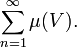$\sum_{n=1}^\infty \mu(V).$

We have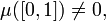$\mu([0,1])\ne0,$ since otherwise$\mu(\mathbb{R}) = \sum_{n=1}^\infty \mu([n,n+1]) = 0.$

Since W contains the interval [0,1] we clearly have$\mu(W) \ge \mu([0,1]),$ which implies that μ(V) is strictly positive. At the same time, the infinite sum is bounded by$\mu([-1,2]) < \infty,$ which is a contradiction. Consequently,$V\notin \Sigma.$Some content on this page may previously have appeared on Citizendium.